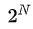Propeller 2 NCO (Numerically Controlled Oscillator)

• Each P2 I/O pin can be used as an NCO to create a square wave output at virtually any frequency up to half the operating frequency
• There are four instructions needed to do this wrpin, wxpin, wypin and drvh (drvh has to be last).
• in FlexProp C using inline assembly to create NCO on pin #8 (12.375 MHz with 297 MHz P2 clock):
•   __asm {
wrpin ## 0b00000000000000000000000011001100, #8               //smartpin NCO
WXPIN #12, #8                                                                          //base period
WYPIN ##\$8000_0000, #8                                                   //add amount per clock
drvh #8
}
• In Spin2 using inline assembly:
•  CON 'NCO
' %AAAA_BBBB_FFF_PPPPPPPPPPPPP_TT_MMMMM_0
pinset3 = %0000_0000_000_0000000000000_11_00110_0 'NCO smartpin mode
• org
'NCO on pin #8
wrpin ##pinset3,#8 'smartpin
WXPIN #12,#8 ' 'base period
WYPIN ##\$0020_0000,#8 'add amount per clock
drvh #8
END
• You can avoid assembly using special methods in Spin2 and C.
•  In Spin2, use WRPIN(), WXPIN(), WYPIN(), and PINH()
• In FlexProp C, use _wrpin(), _wxpin(), _wypin(), and _pinh()
• See Propeller2.h for details
• Code can be simplified using predefined constants and also the PINSTART instruction
• The above can be made clearer with some predefined constants:   wrpin ##(P_OE | P_NCO_FREQ), #8
• The four instructions can be combined into one with PINSTART:
•  SPIN2:  PINSTART(8, (P_OE | P_NCO_FREQ), 12, \$8000_0000)
•  FlexC:  _pinstart(8, P_NCO_FREQ+P_OE, 12, 0x8000_0000);
• The output frequency can be determined with the help of some equations from Wikipedia
• Here is the basic equation:• ΔF is the # added to accumulator every P2 clock tick.  For P2, this is the WYPIN value divided by the WXPIN value.  In the case of the code above, this is \$8000_0000/12 (0x80000000/12) = 2,147,483,648/12 = 178,956,970
• The P2 is slightly different from what is giving in Wikipedia in that we have the extra WXPIN value that slows down the accumulator.  You could think of it as dividing the P2 clock frequency by this value.
• The P2 accumulator is 32 bits, so= 2^32 = 4,294,967,296
• Fclock is the P2 clock frequency.
• So, the code above with a P2 clock of 297 MHz produces an NCO frequency of  (297 MHz)* 178,956,970 / 4,294,967,296  = 12.375 MHZ